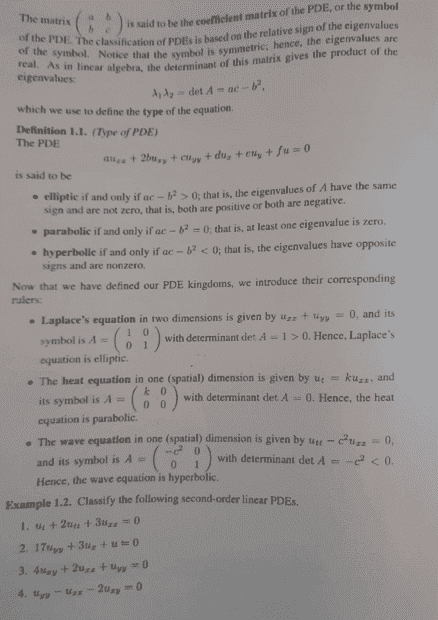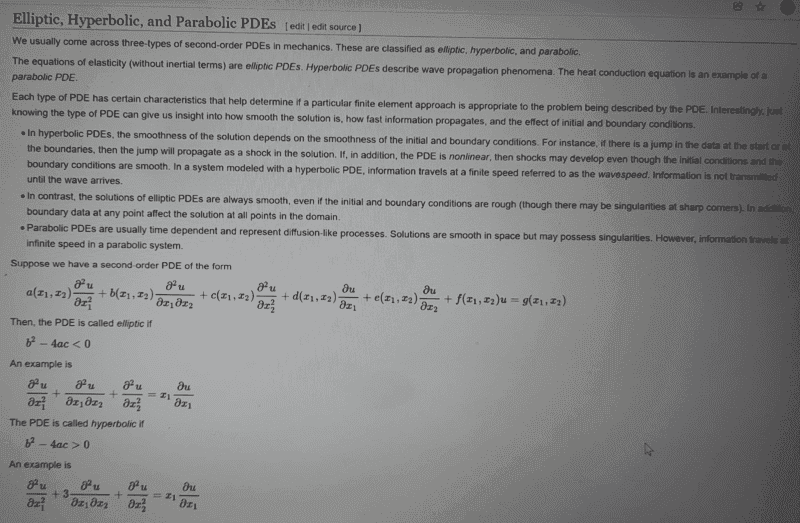# Classify the given second-order linear PDE

Gold Member
Homework Statement:
Classify the second order linear pde, given by;

##U_t +2U_{tt}+3U_{xx}=0##
Relevant Equations:
use of discriminant
Now i learned how to use discriminant i.e ##b^2-4ac## and in using this we have;
##b^2-4ac##=##0-(4×3×2)##=##-24<0,## therefore elliptic.

The textbook has a slight different approach, which i am not familiar with as i was trained to use the discriminant at my undergraduate studies...
see textbook approach here;and the textbook solution is here;and i also checked wikipedia on this and they have used the discriminant approach. See the approach here; ( i am comfortable with this approach)Both approaches should be fine, one can use either approach...correct?

Staff Emeritus
Gold Member
The textbook is the same thing with some math modifications

1.) Instead of ##b^2-4ac## they have ##4ac-b^2## so the sign is flipped

2.) They write ##2b## as the coefficient instead of ##b##. So given that the coefficient of the cross term is called ##2b##, their discriminant becomes
##4ac-(2b)^2=4(ac-b^2)##. Since 4 is positive, the sign of the discriminant is determined by ##ac-b^2##.

•sysprog
Gold Member
The textbook is the same thing with some math modifications

1.) Instead of ##b^2-4ac## they have ##4ac-b^2## so the sign is flipped

2.) They write ##2b## as the coefficient instead of ##b##. So given that the coefficient of the cross term is called ##2b##, their discriminant becomes
##4ac-(2b)^2=4(ac-b^2)##. Since 4 is positive, the sign of the discriminant is determined by ##ac-b^2##.
ooooh, Thanks am trying to refresh on pde's cheers mate...